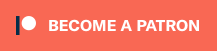## partial_function

A clean way to define function as a set of subfunctions where each has defined start and end bounds

### 7 releases(4 breaking)

 0.5.0 May 24, 2020 Aug 9, 2018 Aug 8, 2018 Sep 27, 2017 Sep 27, 2017

#525 in Rust patterns

Used in 2 crates

Apache-2.0

10KB
115 lines

Support an Open Source Developer! ♥️# Partial Function

A clean way to define function as a set of smaller functions where each has defined start and end bounds.

## Partial Function

Achieves the following:

``````f(x) = {
x     if 0 <= x <   5
x * 2 if 5 <= x <= 10
}
``````

Expressed as:

``````let p = PartialFunction::new()
.with(0.0, 5.0,  Box::new(|x| x    ))
.with(5.0, 10.0, Box::new(|x| x * 2))
.build();
assert_eq!(p.eval(5.0), Some(10.0));
``````

## Lower Partial Function

Achieves the following:

``````f(x) = {
x     if 0 <= x <   5
x * 2 if 5 <= x
}
``````

Expressed as:

``````let f = LowerPartialFunction::new()
.with(0.0, Box::new(|x| x    ))
.with(5.0, Box::new(|x| x * 2))
.build();
assert_eq!(f.eval(5.0), Some(10.0));
``````

~220–630KB
~15K SLoC